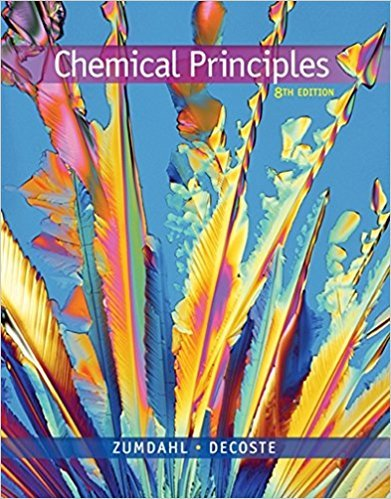×
Get Full Access to Chemical Principles - 8 Edition - Chapter 2 - Problem 2.24
Get Full Access to Chemical Principles - 8 Edition - Chapter 2 - Problem 2.24

×

# Consider 80.0-g samples of two different compoundsISBN: 9781305581982 176

## Solution for problem 2.24 Chapter 2

Chemical Principles | 8th Edition

• Textbook Solutions
• 2901 Step-by-step solutions solved by professors and subject experts
• Get 24/7 help from StudySoup virtual teaching assistantsChemical Principles | 8th Edition

4 5 1 329 Reviews
26
3
Problem 2.24

Consider 80.0-g samples of two different compounds consisting of only carbon and oxygen. One of the compounds consists of 21.8 g of carbon, and the other has 34.3 g of carbon. Determine the ratio in whole numbers of the masses of carbon that combine with 1.00 g of oxygen between the two compounds.

Step-by-Step Solution:

Problem 2.24

Consider 80.0-g samples of two different compounds consisting of only carbon and oxygen. One of the compounds consists of 21.8 g of carbon, and the other has 34.3 g of carbon. Determine the ratio in whole numbers of the masses of carbon that combine with 1.00 g of oxygen between the two compounds.

Step by Step Solution

Step 1 of 2

Compound -1:

From the given,

Total mass of both compounds = 80.0 g

The mass of carbon = 21.8 g

The mass of carbon that combines with one gram of oxygen.

Let’s calculate the mass of carbon per one gram of oxygen.Compound -2:

From the given,

Total mass of both compounds = 80.0 g

The mass of carbon = 34.3 g

The mass of carbon that combines with one gram of oxygen.

Let’s calculate the mass of carbon per one gram of oxygen.Step 2 of 2

##### ISBN: 9781305581982

Unlock Textbook Solution